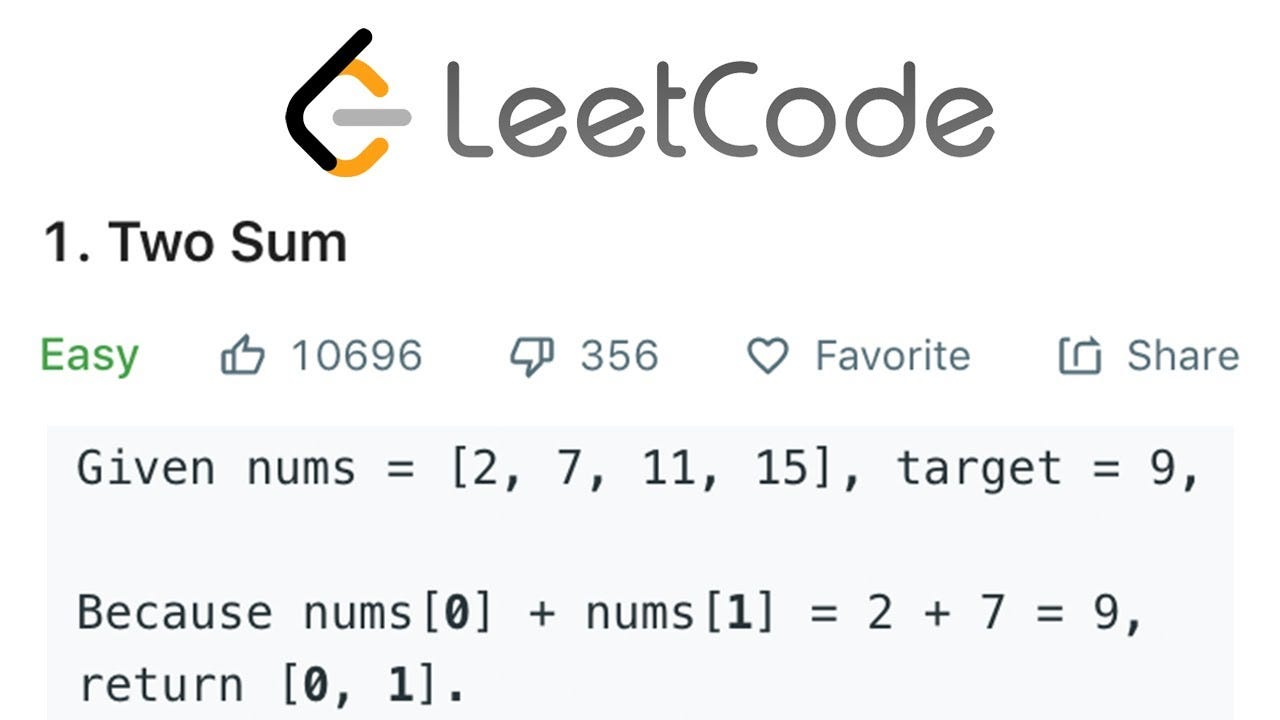# Solution: Two Sum

## You’re given an array of integers. Write a function that returns a pair of numbers such that they sum up to zero.[Alternate problem with a target]

You can assume there will be exactly 1 solution. Each element in the array can only be used once.

Example:

`Array of integers is [2, 7, 9, -2].The pair that sums up to 0 is (2, -2).`

Input:
A list of numbers, separated by space.

`2 7 9 -2`

Output:
2 numbers from the array that sum up to 0.
We will sort the 2 numbers for you so that you can easily compare with our expected output.

`-2 2`

# Observe

1. For a number A, we want to know if such a number B exists, such that A + B = 0.

Insights:
A brute force approach of 2 nested for loops, one to find A and one to find B should solve the problem, but it is highly inefficient, at O(N²) complexity.

2. Notice that B = -A.

Insights:
Instead of making a 2nd nested for loop to find B, we can use a hash map to quickly check if B exists in the array.

# Algorithm

1. Create an empty hash map to store the occurrence count for each number in the array.
2. Iterate through the array.
For each number A, check if -A is in the hash map.
If so, return the pair (A, -A). Otherwise, put A in the hash map.

# Code

`/** * @param {Array<Number>} nums * @param {Array<Number>} 2 numbers from nums that add up to 0. */function pairOfZeroSum(nums) {  const hashmap = new Map();  for (let i = 0; i < nums.length; i++) {    if (hashmap.has(-nums[i])) {      return [nums[i], -nums[i]];    }    hashmap.set(nums[i], true);  }  // We should not reach this point since a solution is guaranteed  // to exist.  return [];}`

# Complexity Analysis

We visit each number in the array only once. At each visit, we call a couple of hashmap functions, each of which is at O(1) complexity.

Hence, the overall complexity is O(N).

Software Engineer @Vedantu, Former Intern @Hackerrank, GSoC @Wikimedia, Codeforces(Expert)

## More from S7rthak

Software Engineer @Vedantu, Former Intern @Hackerrank, GSoC @Wikimedia, Codeforces(Expert)